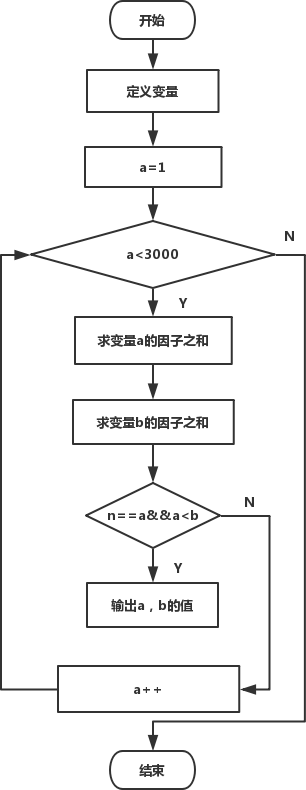• 内容
• 评论
• 相关

## 算法设计```#include<stdio.h>
int main()
{
int a, i, b, n;
printf("There are following friendly--numbers pair smaller than 3000:\n");
for( a=1; a<3000; a++ )  /*穷举3000以内的全部整数*/
{
for( b=0, i=1; i<=a/2; i++)  /*计算数a的各因子，各因子之和存放于b*/
if(!(a%i))
b+=i;
for( n=0, i=1; i<=b/2; i++ )  /*计算b的各因子，各因子之和存于n*/
if(!(b%i))
n+=i;
if(n==a && a<b)  /*使每对亲密数只输出一次*/
printf("%4d--%4d    ", a, b);  /*若n=a，则a和b是一对亲密数，输出*/
}

return 0;
}```

There are following friendly--numbers pair smaller than 3000:
220-- 284    1184--1210    2620--2924

0条评论